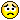# Help me -- meprec problem

Posted 10 years ago
10862 Views
|
6 Replies
|
3 Total Likes
|
 I have been stuck here for almost two weeks! My input is too big and my Mathematica can't run the program fully, yet the result is not the one that I want. can someone help me?I inserted this kind of precision code and already play around with the coding.Block[{$MaxExtraPrecision = 5000}, Table[N[{*my very long inputs. 4 of them*}],{x,0,2Pi,Pi/90}]]and the output that I have is thisN::meprec: "Internal precision limit$MaxExtraPrecision = 5000. reached while evaluating Log[Cos[\[Pi]/30]^2+Sin[\[Pi]/30]^2]."N::meprec: "Internal precision limit $MaxExtraPrecision = 5000. reached while evaluating Log[Cos[\[Pi]/30]^2+Sin[\[Pi]/30]^2]."N::meprec: "Internal precision limit$MaxExtraPrecision = 5000. reached while evaluating Log[Cos[\[Pi]/30]^2+Sin[\[Pi]/30]^2]."General::stop:Further output of N::meprec will be suppressed during this calculation. What should I do?6 Replies
Sort By:
Posted 10 years ago
 To simplify your example,In:= N[Log[Cos[\[Pi]/30]^2 + Sin[\[Pi]/30]^2], 100]During evaluation of In:= N::meprec: Internal precision limit \$MaxExtraPrecision = 50. reached while evaluating Log[Cos[\[Pi]/30]^2+Sin[\[Pi]/30]^2]. >>Out= 0.*10^-151You have a hidden zero. Mathematica raises precision in an effort to find significant digits. There are none to be found. Eventually mathematica picks up its marbles and leaves.
Posted 10 years ago
 Can you find a free service where you can upload your notebook of four long lines and post where that is so that others can download the notebook without having to sign up, register, log in, etc?Can you tell how how many digits of precision you need to have in your four results?With that notebook and the needed precision perhaps someone can find a way for you to get your results.
Posted 10 years ago
 Yes. The inputs are the roots polynomials. I'm not sure about this yet but I'll try it. Then it is possible to combine back the answers?Sorry too much question to ask, I'm new in Mathematica.
Posted 10 years ago
 Your post says you have four very long inputs.  Try running them one at a time.You may be running out of RAM and using the hard drive, which is very slow.
Posted 10 years ago
 Can you split it up into smaller parts?
Posted 10 years ago
 Split? Do you mean that I have to run the code separately?